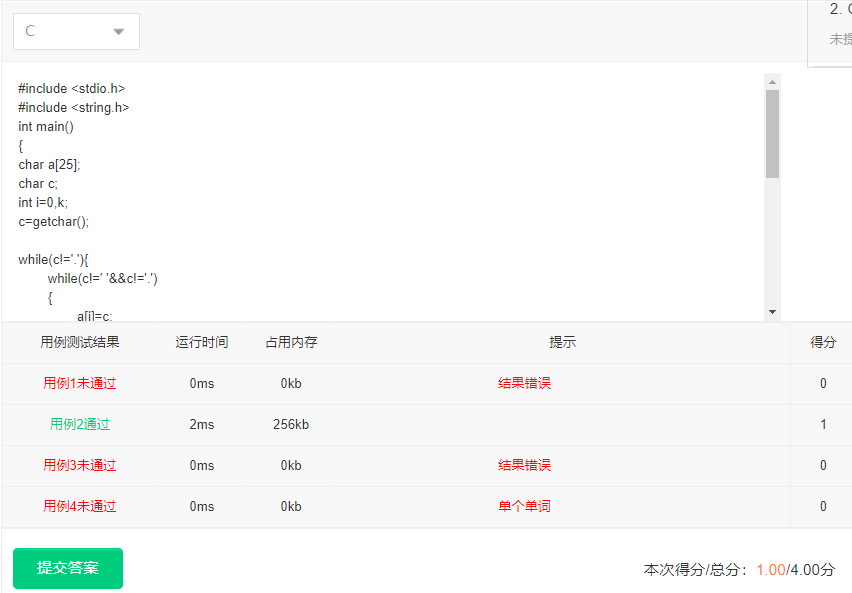2021-11-02 19:38

# c语言用getchar函数取得单词长度时碰到的问题

It's great to see you here.

4 5 2 3 3 4``````#include <stdio.h>
#include <string.h>
int main()
{
char a;
char c;
int i=0,k;
c=getchar();

while(c!='.'){
while(c!=' '&&c!='.')
{
a[i]=c;
i++;
c=getchar();
}
if(c!='.')
{
printf("%d ",strlen(a));
i=0;
for(k=0;k<25;k++)
{
a[k]='\0';
}
c=getchar();
}else {
printf("%d",strlen(a));
}
}
return 0;
}

``````
• 写回答
• 好问题 提建议
• 追加酬金
• 关注问题
• 邀请回答